# Frequency school and Bayes school

Frequentists think that , The distribution parameter to which the sample belongs θ Although unknown , But it's fixed , It's possible to sample $θ$ Make an estimate to get $\theta{\hat{}}$ . Bayesian school thinks that parameters $θ$ It's a random variable , It's not a fixed value , Before the sample is produced , Will be based on experience or other methods to θ Preset a distribution $\pi(\theta)$, It's called a priori distribution . After that, we'll make a comparison of $θ$ Adjustment , correct , Write it down as $\pi(\theta|x1,x2,x3,……)$, It's called posterior distribution .

# The derivation of Bayes formula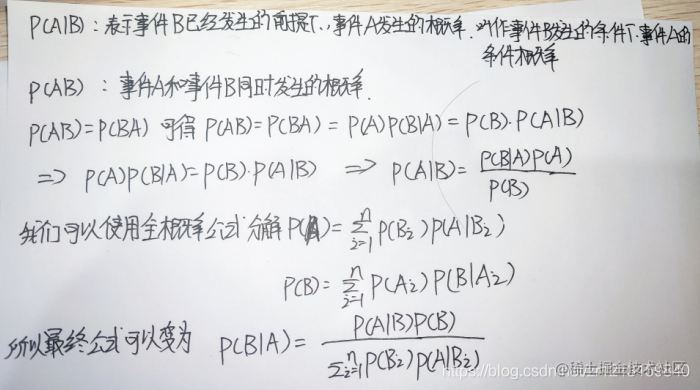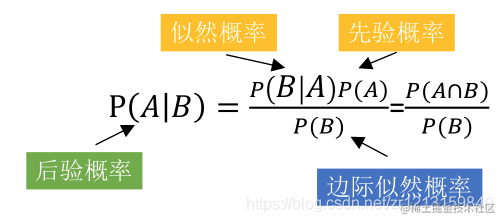# Why naive Bayes

Suppose that the attributes of the training data are determined by n Dimension random vector x Express , The results of classification are random variables y Express , that x and y We can use the joint probability distribution P ( X , Y ) P(X,Y)P(X,Y) describe , Each specific sample ( x i , y i ) (x_i,y_i)(x i ​ ,y i ​ ) Both can pass P ( X , Y ) P(X,Y)P(X,Y) Independent identically distributed generation The starting point of Bayesian classifier is joint probability distribution , According to the properties of conditional probability, we can get P ( X , Y ) = P ( Y ) ∗ P ( X ∣ Y ) = P ( X ) ∗ P ( Y ∣ X ) P(X,Y)=P(Y)*P(X|Y)=P(X)*P(Y|X)P(X,Y)=P(Y)∗P(X∣Y)=P(X)∗P(Y∣X) among P ( Y ) P(Y)P(Y)： Probability of occurrence of each category , This is a priori probability . P ( X ∣ Y ) P(X|Y)P(X∣Y)： The probability of different attributes in a given category , Likelihood probability A priori probability is easy to calculate , Just count the number of samples of different categories , The likelihood probability is affected by the number of attributes , It is difficult to estimate . for example , Each sample contains 100 Attributes , The value of each attribute may have 100 Kind of , Every result of that classification , The conditional probability to calculate is 1002=10000, The amount is huge . therefore , At this time, naive Bayes was introduced .

# What is naive Bayes

Naive Bayes , Added a simple , It means simpler Bayes . Naive Bayes assumes that different attributes of samples satisfy the conditional independence hypothesis , On this basis, Bayes theorem is applied to perform the task of classification . For a given item to be classified x, Analyze the posterior probability of the sample in each category , Take the class with the greatest posterior probability as x Category To solve the problem that likelihood probability is difficult to estimate , We need to introduce conditions Independence hypothesis The conditional independence assumption guarantees that all attributes are independent of each other , They don't influence each other , Each attribute has an independent effect on the classification results . In this way, the conditional probability becomes the product of attribute conditional probability $P(X = x|Y = c) = P(X(1)=x(1),X(2)=x(2),……,X(n)=x(n)|Y=c)=\ i=0∏n​P(Xj=xj∣Y=c)$ This is the naive Bayes method , With the training set , We can easily work out a priori probability P ( Y ) P(Y)P(Y) And likelihood probability P ( Y ∣ X ) P(Y|X)P(Y∣X), So we can get the posterior probability P ( X ∣ Y ) P(X|Y)P(X∣Y)

# Example – Watermelon book 151 page

First of all, we have a dataset of watermelon 3.0.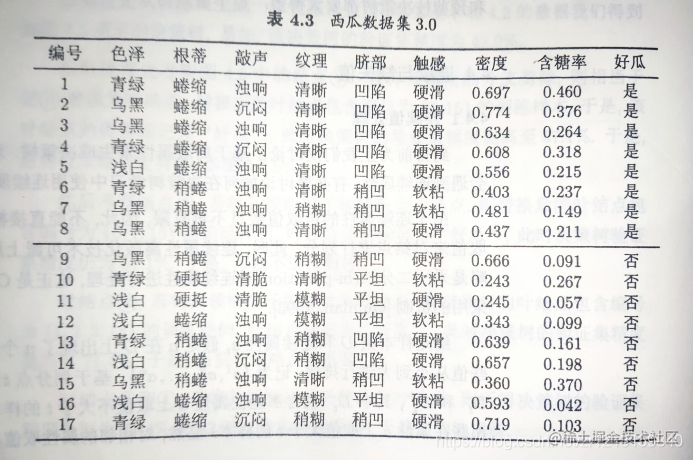We have a problem . The following test set is good or bad ？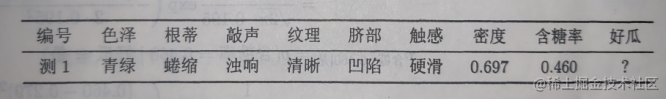We can first calculate a priori probability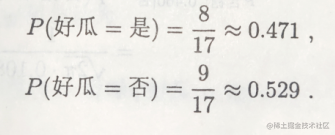And then calculate the conditional probability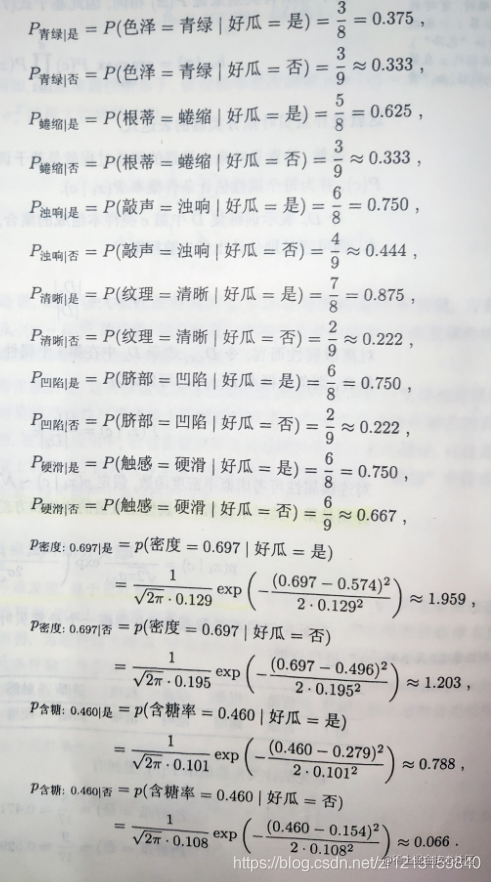Then calculate the probability of good and bad melons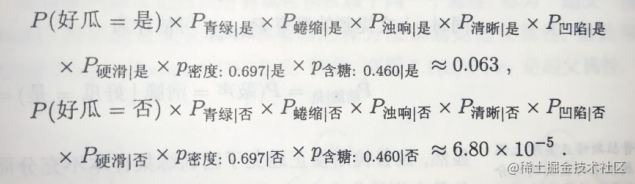0.063 Significantly larger , So it's probably a good melon .

https://cdmana.com/2021/07/20210718125051383D.html

Scroll to Top